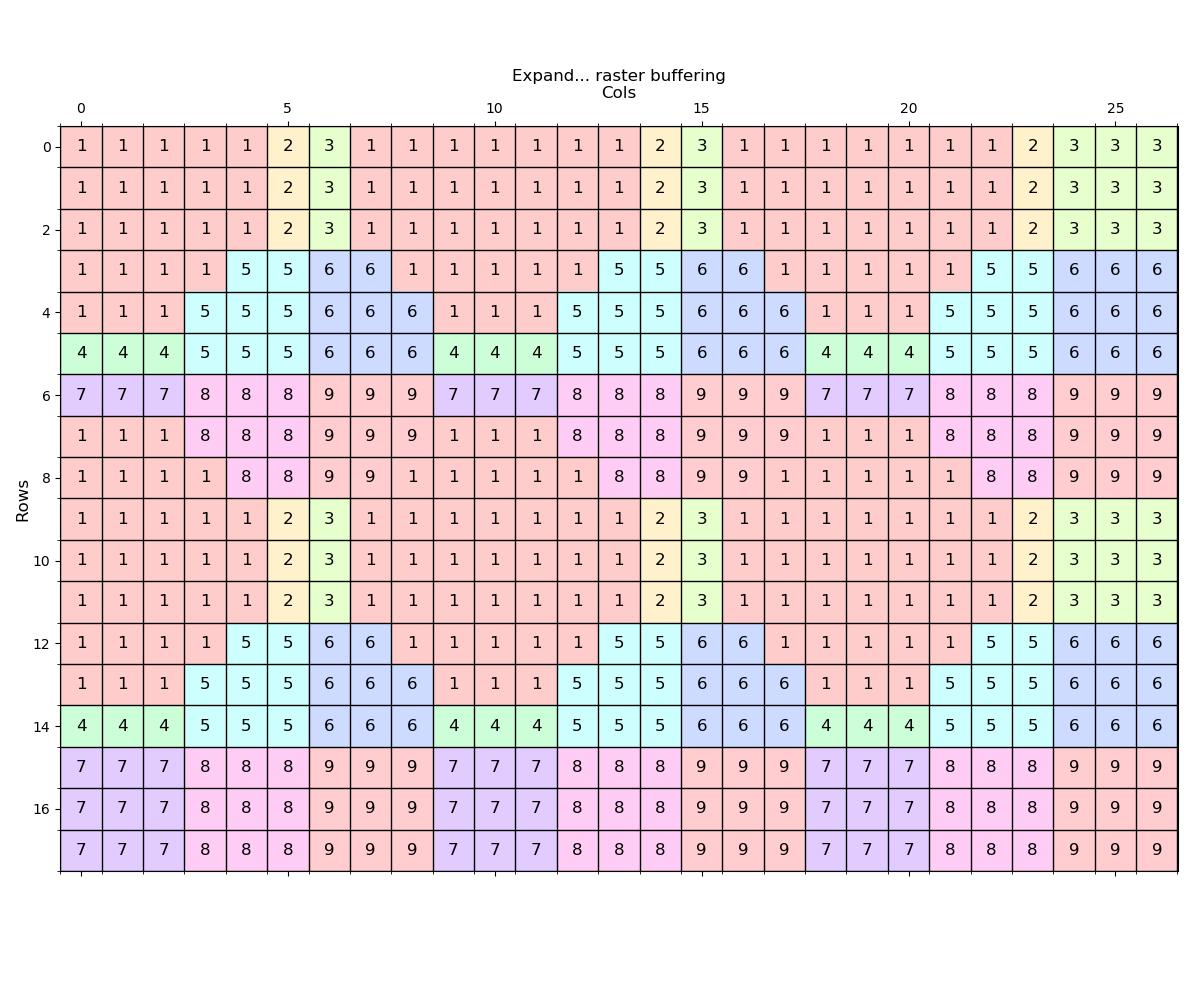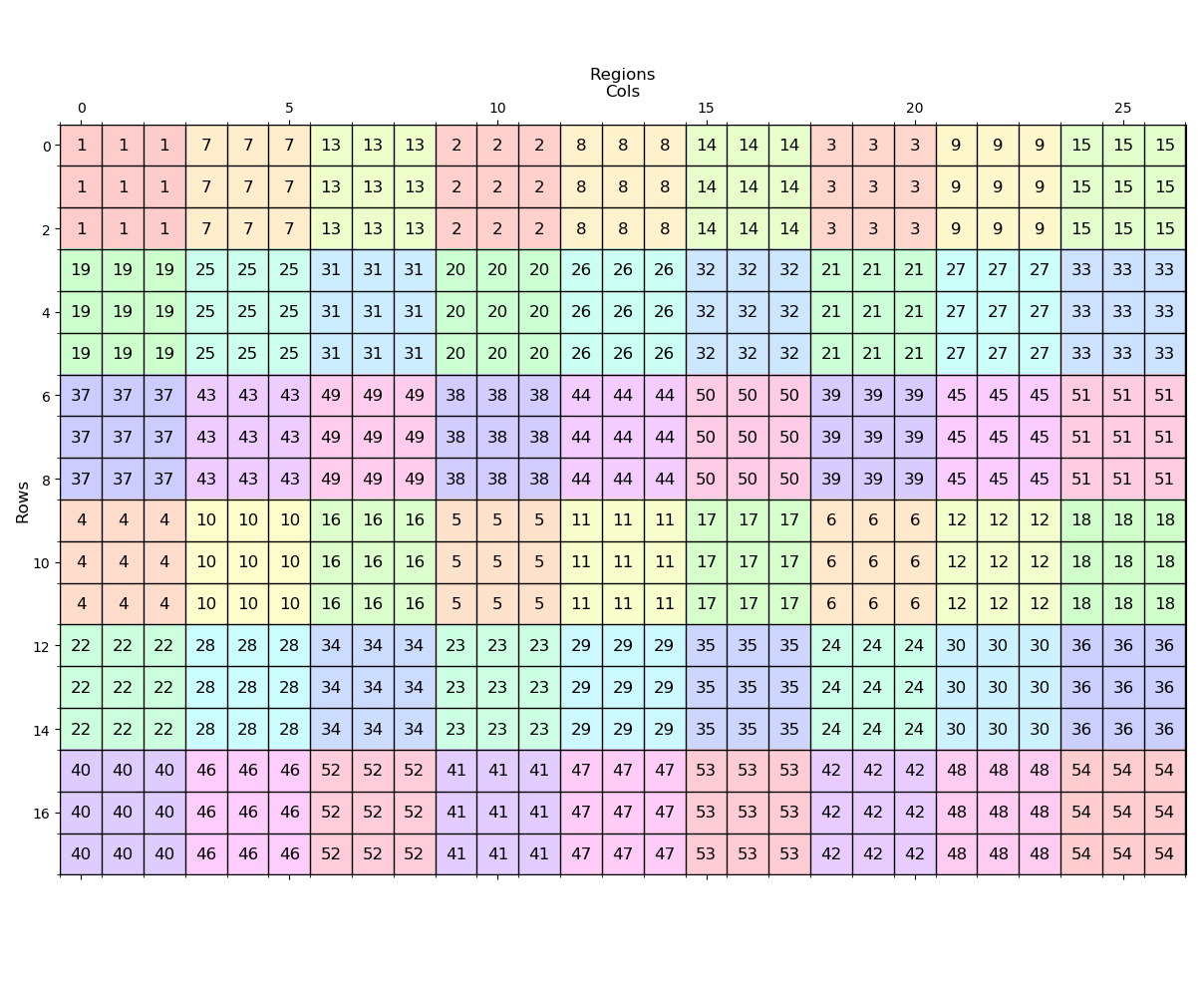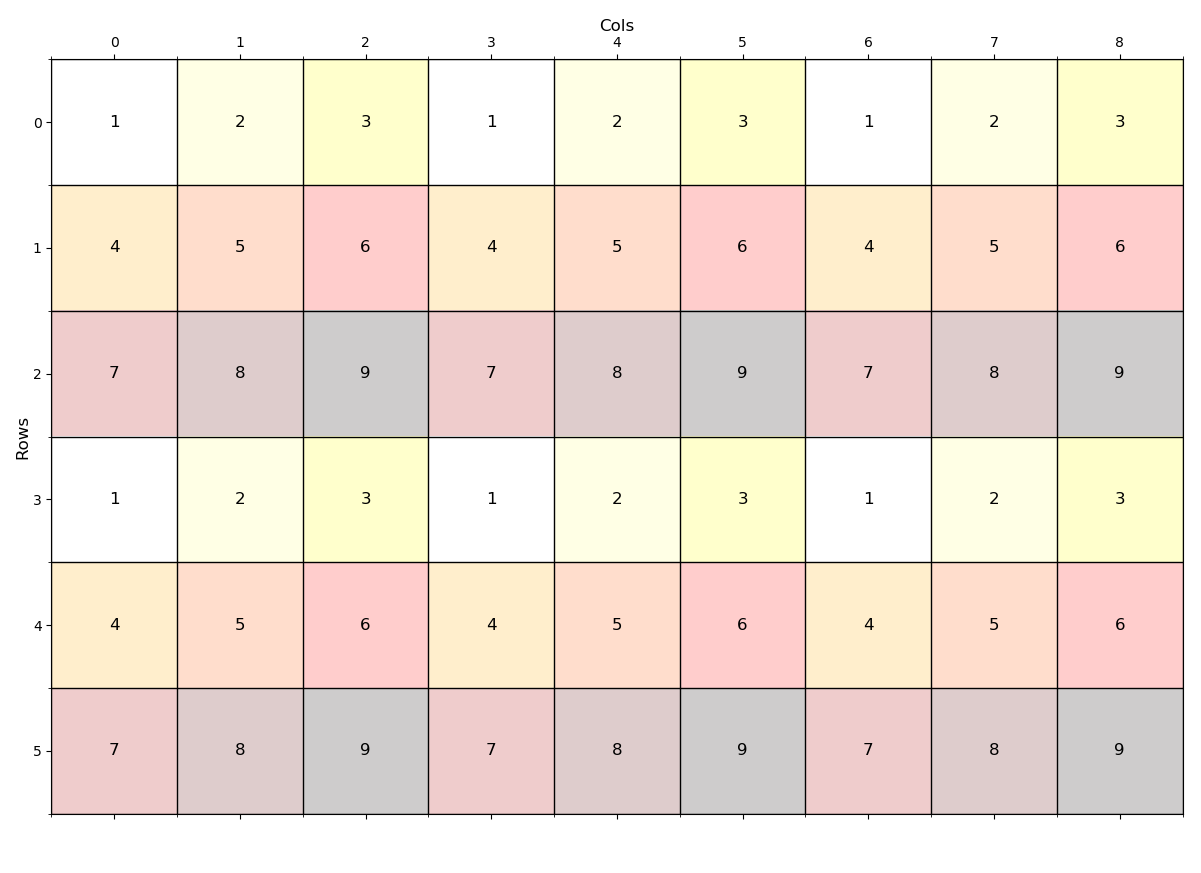# Generalization tools for rasters...

797
0
10-08-2018 03:14 AM
Labels (1)byMVP Esteemed Contributor
0 0 797

Scipy ndimage morphology … I am betting that is the first thought that popped into your head.  A new set of tools for the raster arsenal.

But wait!  Their terminology is different than that used by ArcGIS Pro or even ArcMap.

What tools are there?  Lots of filtering tools, but some of the interesting ones are below

``from scipy import ndimage as nddir(nd)[... snip ... 'affine_transform', ... , 'center_of_mass', 'convolve', 'convolve1d', 'correlate', 'correlate1d', ... 'distance_transform_edt', ... 'filters', ... 'generic_filter', ... 'geometric_transform', ... 'histogram', 'imread', 'interpolation', ... 'label', 'labeled_comprehension', ... 'measurements', ... 'morphology', ...  'rotate', 'shift', 'sobel',...]‍‍‍‍‍‍‍‍‍‍‍‍‍‍‍‍‍‍‍‍‍‍‍‍‍‍‍‍‍‍‍‍‍‍‍‍‍‍‍‍‍‍‍‍‍‍‍‍‍‍‍‍‍‍``

I previously covered distance_transform_edt which performs the equivalent of Euclidean Distance and Euclidean Allocation in this post

Let's begin with a raster constructed from 3x3 windows with a repeating pattern and repeats of the pattern.

You can construct your own rasters using numpy, then apply a NumPyArrayToRaster to it.  I have covered this before, but here is the code to produce the raster

``def _make_arr_():    """Make an array with repeating patterns    """    a = np.arange(1, 10).reshape(3, 3)    aa = np.repeat(np.repeat(a, 3, axis=1), 3, axis=0)    aa = np.tile(aa, 3)    aa = np.vstack((aa, aa))    return aa‍‍‍‍‍‍‍‍‍‍‍‍‍‍‍‍‍‍‍‍‍‍‍‍``

---- The raster (image) -------- (1) Expand ----

Expand the zone 1 values by 2 distance units---- (2) Shrink ----

Shrink the zone 1 values by 1 distance unit.---- (3) Regiongroup ----

Produce unique regions from the zones.  observe that the zone 1 values in the original are given unique values first. Since there were 6 zone 1s, they are numbered from 1 to 6.  Zone 2 gets numbered from 7 to 12.  and that pattern of renumbering repeats.---- (4) Aggregate ----

Aggregation of the input array using the mode produces a spatial aggregation retaining the original values.  Our original 3x3 kernels are now reduced to a 1x1 size which has 3 times the width and height (9x area).---- (5) Some functions to perform the above ----

In these examples buff_dist is referring to 'cells'.

For the aggregation, there are a number of options as shown in the header.

Generally integer data should be restricted to the mode, min, max, range and central (value) since the median and mean upscale to floating point values.  This of course can be accommodated by using the python statistics package median_low and median_high functions:

So think of a function that you want.  Filtering is a snap since you can 'stride' an array using any kernel you want using plain numpy or using the builtin functions from scipy.

Enough for now...

``def expand_(a, val=1, mask_vals=0, buff_dist=1):    """Expand/buffer a raster by a distance    """    if isinstance(val, (list, tuple)):        m = np.isin(a, val, invert=True).astype('int')    else:        m = np.where(a==val, 0, 1)    dist, idx = nd.distance_transform_edt(m, return_distances=True,                                          return_indices=True)    alloc = a[tuple(idx)]    a0 = np.where(dist<=buff_dist, alloc, a)  #0)    return a0def shrink_(a, val=1, mask_vals=0, buff_dist=1):    """Expand/buffer a raster by a distance    """    if isinstance(val, (list, tuple)):        m = np.isin(a, val, invert=False).astype('int')    else:        m = np.where(a==val, 1, 0)    dist, idx = nd.distance_transform_edt(m, return_distances=True,                                          return_indices=True)    alloc = a[tuple(idx)]    m = np.logical_and(dist>0, dist<=buff_dist)    a0 = np.where(m, alloc, a)  #0)    return a0def regions_(a, cross=True):    """currently testing regiongroup    np.unique will return values in ascending order    """    if (a.ndim != 2) or (a.dtype.kind != 'i'):        msg = "\nA 2D array of integers is required, you provided\n{}"        print(msg.format(a))        return a    if cross:        struct = np.array([[0,1,0], [1,1,1], [0,1,0]])    else:        struct = np.array([[1,1,1], [1,1,1], [1,1,1]])    #    u = np.unique(a)    out = np.zeros_like(a, dtype=a.dtype)    details = []    is_first = True    for i in u:        z = np.where(a==i, 1, 0)        s, n = nd.label(z, structure=struct)        details.append([i, n])        m = np.logical_and(out==0, s!=0)        if is_first:            out = np.where(m, s, out)            is_first = False            n_ = n        else:            out = np.where(m, s+n_, out)            n_ += n    details = np.array(details)    details = np.c_[(details, np.cumsum(details[:,1]))]    return out, detailsdef aggreg_(a, win=(3,3), agg_type='mode'):    """Aggregate an array using a specified window size and an aggregation    type    Parameters    ----------    a : array        2D array to perform the aggregation    win : tuple/list        the shape of the window to construct the blocks    agg_type : string aggregation type        max, mean, median, min, mode, range, sum, central    """    blocks = block(a, win=win)    out = []    for bl in blocks:        b_arr = []        for b in bl:            if agg_type == 'mode':                uni = np.unique(b).tolist()                cnts = [len(np.nonzero(b==u)) for u in uni]                idx = cnts.index(max(cnts))                b_arr.append(uni[idx])            elif agg_type == 'max':                b_arr.append(np.nanmax(b))            elif agg_type == 'mean':                b_arr.append(np.nanmean(b))            elif agg_type == 'median':                b_arr.append(np.nanmedian(b))            elif agg_type == 'min':                b_arr.append(np.nanmin(b))            elif agg_type == 'range':                b_arr.append((np.nanmax(b) - np.nanmin(b)))            elif agg_type == 'sum':                b_arr.append(np.nansum(b))            elif agg_type == 'central':                b_arr.append(b.shape//2, b.shape//2)            else:                tweet("\naggregation type not found {}".format(agg_type))                b_arr.append(b.shape//2, b.shape//2)        out.append(b_arr)    out = np.array(out)    return out‍‍‍‍‍‍‍‍‍‍‍‍‍‍‍‍‍‍‍‍‍‍‍‍‍‍‍‍‍‍‍‍‍‍‍‍‍‍‍‍‍‍‍‍‍‍‍‍‍‍‍‍‍‍‍‍‍‍‍‍‍‍‍‍‍‍‍‍‍‍‍‍‍‍‍‍‍‍‍‍‍‍‍‍‍‍‍‍‍‍‍‍‍‍‍‍‍‍‍‍‍‍‍‍‍‍‍‍``